# Solving Ordinary Differential Equations

Summation of solutions to ODEs.

An ordinary differential equation (ODE) is an equation that contains one or several derivatives of an unknown function.

ADVANCED ENGINEERING MATHEMATICS - ERWIN KREYSZIG

• The ORDER of an ODE is defined as the degree of the highest derivative in the equation.
• An ODE is LINEAR if the dependent variable and its derivatives do not appear in products with themselves, raised to powers or in nonlinear functions. e.g. $\dot{y}+5y=cos(x)\rightarrow \text{$1^{st}$order, linear}$ but $\dot{y}+5y=cos(y)\rightarrow \text{$1^{st}$order, nonlinear}$.
• Put the dependent variable and its derivatives on the left-hand side of the equation.
• RHS=0: Homogeneous ODEs.

## Principles to solve ODEs

• Direct inspection
• Separation of variable
• Integrating factors

### Direct inspection

• Exponential, e.g. $$\frac{dx}{dt}=-4x\Rightarrow x(t)=Ce^{-4t}$$

• Sinusoidal, e.g. $$\ddot{x}+\lambda^2x=0\Rightarrow x(t)=sin(\lambda t)\text{ or }cos(\lambda t)$$

### Separable ODEs

Given an ODE: $$\frac{dx}{dt}=f(x,t)$$ Rearrange the equation: $$g(x)\frac{dx}{dt}=h(t)$$ And then do integration: $$\int g(x)dx=\int h(t)dt+C$$

#### Extended method: reduction to separable form

Make form below, and $\dot{x}\gets u$ $$\dot{x}=f(\frac{x}{t})$$

### Exact ODEs

#### Exact case

The ODE's differential form $M(x,t)dx+N(x,t)dt$ is termed EXACT. Say $$M(x,t)\frac{dx}{dt}+N(x,t)=0\\ \frac{\partial u(x,t)}{\partial x}\frac{dx}{dt}+\frac{\partial u(x,t)}{\partial t}=0\\ \frac{\partial u(x,t)}{\partial x}dx+\frac{\partial u(x,t)}{\partial t}dt=0\\ \frac{du}{dt}=0\\ u=C$$ (☞ﾟヮﾟ)☞ $u(x,t)$ or the exactness exists if: $$\frac{\partial M}{\partial t}=\frac{\partial N}{\partial x}$$

#### Non-exact case

##### IF available

Say a non-exact equation: $$P(x,y)dx+Q(x,y)dy=0$$ Use an integrating factor to make the equation exact: $$FPdx+FQdy=0$$ Find the integrating factor: EXACTNESS $$\frac{\partial }{\partial y}(FP)=\frac{\partial }{\partial x}(FQ)\\ F_yP+FP_y=F_xQ+FQ_x\\ \text{Assume the simple cases where F depends on only x (or y)}\\ F=F(x)(\text{ or }F(y))\\ FP_y=F'Q+FQ_x\\ \frac{1}{F}\frac{dF}{dx}=\underset{R}{\frac{1}{Q}(P_y-Q_x)}\\ \text{Assume the simple case where R depends only on x}\\ F(x)=exp\int R(x)dx$$

##### IF unavailable

Choose other methods.

## Solutions to specific forms of ODEs

### 1st-order ODEs

#### Linear cases

$$\dot{x}+p(t)x=r(t)$$

• Homogeneous case: $\dot{x}+p(t)=0$ $$x(t)=A\cdot exp\left(-\int p(t)dt\right)$$

• Non-homogeneous case: $\dot{x}+p(t)x=r(t)$. Apply integrating factor: $$F=exp\left(\int p(t)dt \right)$$

$$x(t)=e^{-\int p(t)dt}\left[\int e^{\int p(t)dt}r(t)dt+C \right]$$

Proof: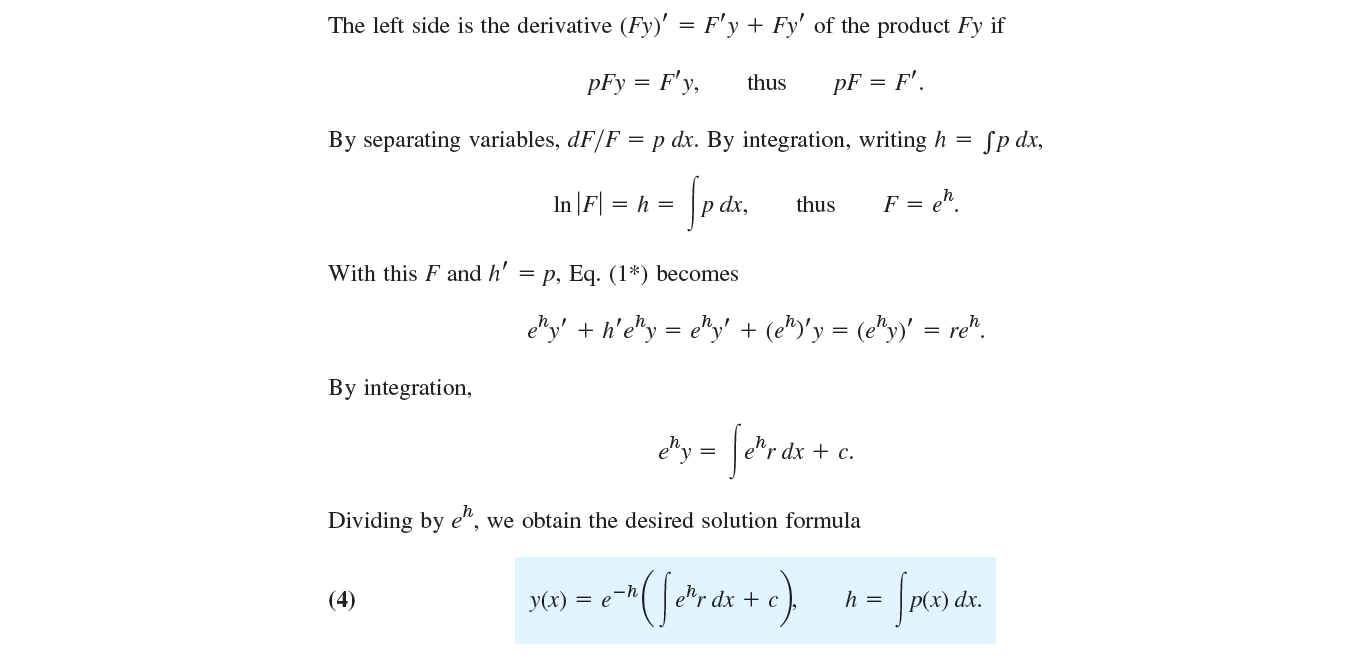#### Non-linear cases: Bernoulli equation

$$\dot{y}+p(x)y=g(x)y^a$$

Let $u(x)=y^{1-a}$, then $$\dot{u}+(1-a)pu=(1-a)g$$

### 2nd-order linear ODEs

$$\ddot{y}+p(x)\dot{y}+q(x)y=r(x)$$

#### Homogeneous cases

##### Linear cases with coefficients as functions

Homogeneous case: $\ddot{y}+p(x)\dot{y}+q(x)y=0$. Superposition principle: any linear combination of two solutions on an open interval is again a solution.

Reduction of order. (when one solution $y_1$ is known). Set $y_2=uy_1$. $$y_2=y_1u=y_1\int \frac{1}{y_1^2}e^{-\int pdx}dx$$

##### Linear cases with constant coefficients

Homogeneous ODE: $$\ddot{y}+a\dot{y}+by=0$$ Characteristic equation (derived from putting $y=e^{\lambda x}$ into the equation): $$\lambda^2+a\lambda+b=0\Rightarrow\lambda_1,\lambda_2$$ Solutions: $y_1=e^{\lambda_1 x}$ and $y_2=e^{\lambda_2 x}$ .

###### Two distinct real roots

Solution: $$y=c_1e^{\lambda_1x}+c_2e^{\lambda_2x}$$

###### Real double root

$$\lambda=\lambda_1=\lambda_2=-\frac{a}{2}$$

Known $y_1=e^{-(a/2)x}$. Apply Reduction of Order: $y_2=uy_1$, then, $$u=c_1x+c_2\Rightarrow u=x\text{ , simple case}$$ Solution: $$y=(c_1+c_2x)e^{-ax/2}$$

###### Complex roots

\begin{aligned} \lambda_1&=-\frac{1}{2}a+i\omega\ \lambda_2&=-\frac{1}{2}a-i\omega \end{aligned}

\begin{aligned} y_1&=e^{-\frac{1}{2}a+i\omega}=e^{-ax/2}cos\omega x\ y_2&=e^{-\frac{1}{2}a-i\omega}=e^{-ax/2}sin\omega x \end{aligned}

Solution: $$y=e^{-ax/2}(Acos\omega x+Bsin\omega x)$$ Properties: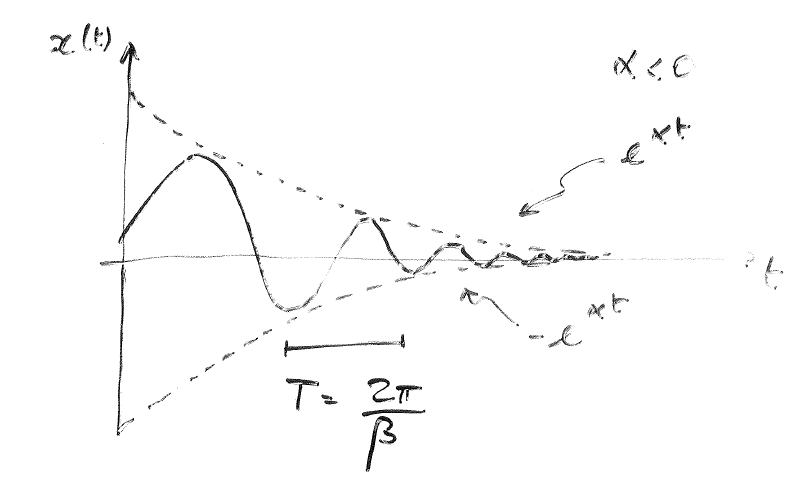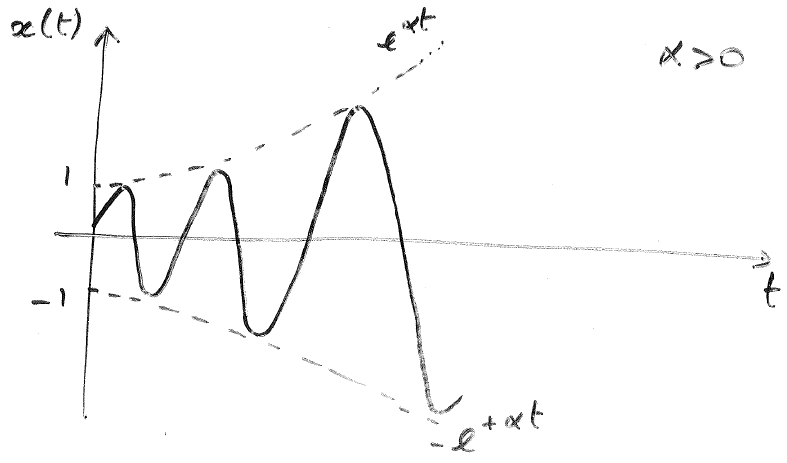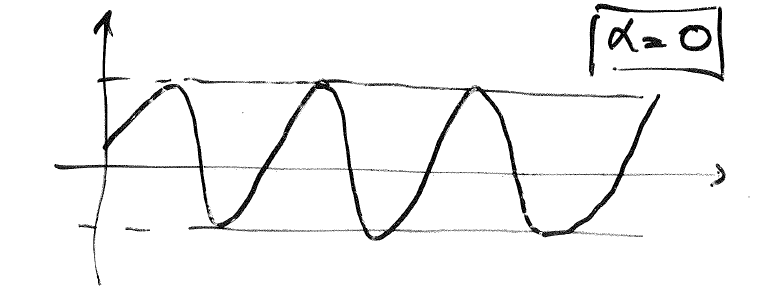Summary in table: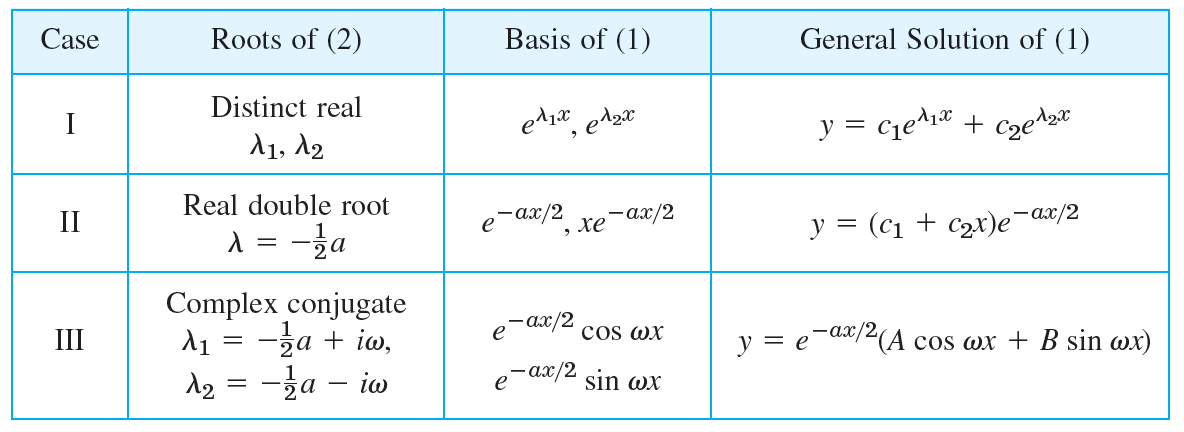#### Non-homogeneous cases

$r(x)\neq 0$. Superposition principle may not exist. $$\ddot{y}+p(x)\dot{y}+q(x)y=r(x)$$ GENERAL SOLUTION: homogeneous solution + particular solution $$y(x)=y_h(x)+y_p(x)$$ Find the PARTICULAR SOLUTION: Method of undetermined coefficients.

• Constant coefficients $$\ddot{y}+a\dot{y}+by=r(x)$$ Choose a form for $y_p$ similar to $r(x)$, but with unknown coefficients to be determined by substituting that $y_p$ and tis derivatives into the ODEs. More rules: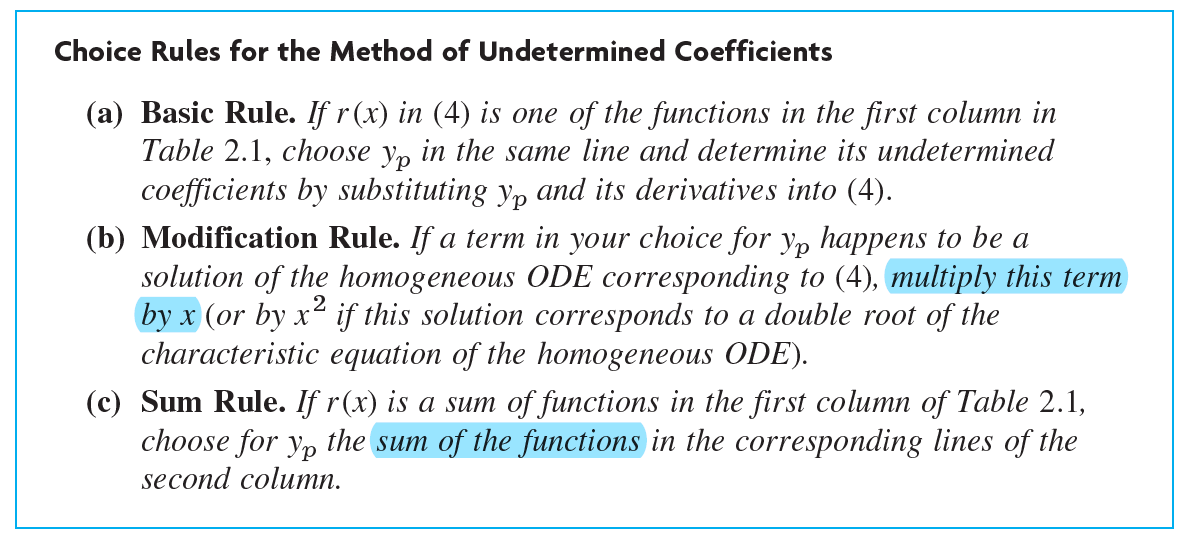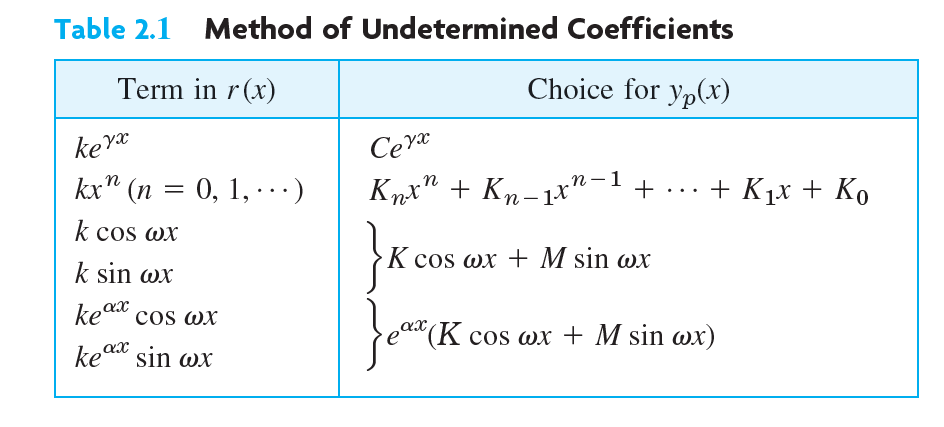first commit